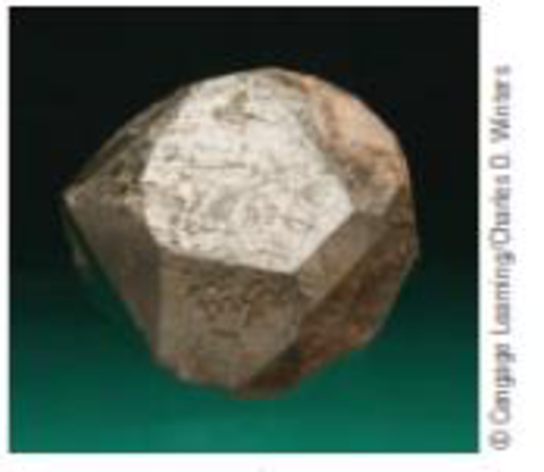Chapter 7, Problem 66IL

Chapter
Section
Textbook Problem

Spinets are solids with the general formula M2+ (M′3+)2O4 (where M2+ and M′3+ are metal cations of the same or different metals). The best-known example is common magnetite, Fe3O4 [which you can formulate as (Fe2+)(Fe3+)2O4].A crystal of a spinet (a) Given its name, it is evident that magnetite is ferromagnetic. How many unpaired electrons are in iron(II) and in iron(III) ions? (b) Two other spinets are CoAl2O4 and SnCo2O4. What metal ions are involved in each? What are their electron configurations? Are the metal ions paramagnetic, and if so, how many unpaired electrons are involved?

a)

Interpretation Introduction

Interpretation:

The number of unpaired electrons present in Iron (II) and Iron (III) has to be predicted.

Concept Introduction:

Electronic configuration: The electronic configuration is the distribution of electrons of an given molecule or respective atoms in atomic or molecular orbital’s. The important there rules for electronic configuration given below.

Aufbau principle: This rule statues that ground state of an atom or ions electrons fill atomic orbitals of the lowest available energy levels before occupying higher levels. If consider the 1s shell is filled the 2s subshell is occupied.

Spinels: This structures are usually cubic close-packed oxides with eight tetrahedral and four octahedral sites per formula unit. The tetrahedral spaces are smaller than the octahedral spaces. B3+ ions occupy half the octahedral holes, while A2+ ions occupy one-eighth of the tetrahedral holes.

Paramagnetic: The Paramagnetic properties are due to the presence of some unpaired electrons, and from the realignment of the electron paths caused by the external magnetic field.

Diamagnetic properties: In diamagnetic materials all the electron are paired so there is no permanent net magnetic moment per atom.

Explanation

Electronic configuration of Fe2O3 molecule

The Iron (Fe2O3) has obeyed for respective electronic configuration for (Fe2+) and (Fe3+) systems:

The electronic configuration of Iron is;

AtomicnumberofIron (Fe)=26spdfwith orbtital notation=[1s22s22p63s23p63d64s2]spdfwith noble gas notation=[Ar]3d64s2Orbitalboxnotation       =  [Ar]3d64s2

Analyzing for Magnetic properties:

• Let us consider the Iron (Fe2+) system:

Iron (Fe)[Ar]3d64s2[AtomicnumberofArgon=18]Loss of two electrons in Feinto Fe2+Orbitalboxnotation       =  [Ar]3d64s0

b)

Interpretation Introduction

Interpretation:

The electronic configuration, paramagnetic property and number of unpaired electrons of the given ions have to be predicted.

Concept Introduction:

Electronic configuration: The electronic configuration is the distribution of electrons of a given molecule or respective atoms in atomic or molecular orbital’s. The important there rules for electronic configuration given below.

Aufbau principle: This rule statues that ground state of an atom or ions electrons fill atomic orbitals of the lowest available energy levels before occupying higher levels. If consider the 1s shell is filled the 2s subshell is occupied.

Spinel: These structures are usually cubic close-packed oxides with eight tetrahedral and four octahedral sites per formula unit. The tetrahedral spaces are smaller than the octahedral spaces. B3+ ions occupy half the octahedral holes, while A2+ ions occupy one-eighth of the tetrahedral holes.

Paramagnetic: The Paramagnetic properties are due to the presence of some unpaired electrons, and from the realignment of the electron paths caused by the external magnetic field.

Diamagnetic properties: In diamagnetic materials all the electron are paired so there is no permanent net magnetic moment per atom.

Still sussing out bartleby?

Check out a sample textbook solution.

See a sample solution

The Solution to Your Study Problems

Bartleby provides explanations to thousands of textbook problems written by our experts, many with advanced degrees!

Get Started

Why are significant figures used?

An Introduction to Physical Science

25-47 What is the purpose of piRNA?

Introduction to General, Organic and Biochemistry

Describe the respiratory mechanism for controlling blood pH.

Chemistry for Today: General, Organic, and Biochemistry